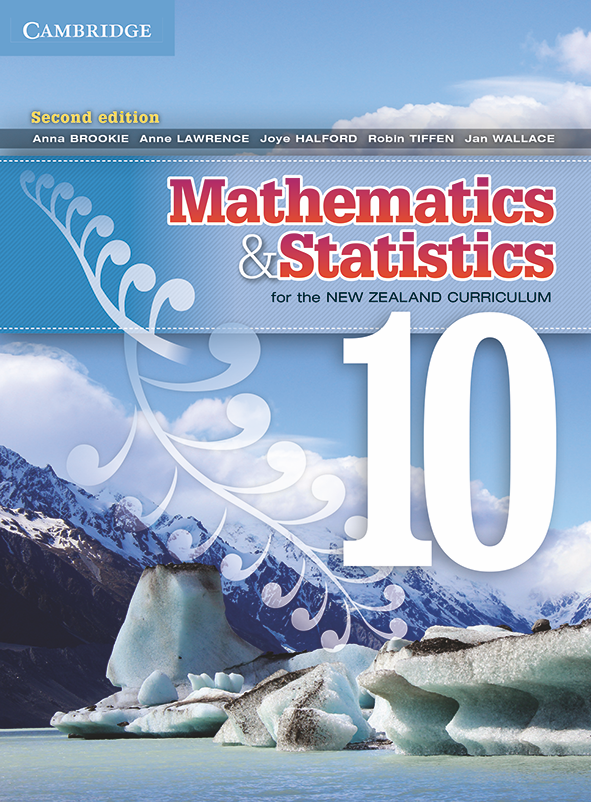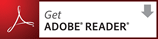## Mathematics and Statistics for the New Zealand Curriculum Year 10 Second Edition

To access your resources ``` Log in ``` or ``` create your Cambridge GO account ``` Activate your resources by entering the access code found in the front of your print textbook, sealed pocket or supplied via email.

Mathematics & Statistics for the New Zealand Curriculum Year 10 2nd Edition provides a strong focus on maths understanding, problem solving and numerical literacy for every student.

PDF Textbook updated 8 November, 2016

To view a sample chapter, click on the file below in blue.

Full PDF Textbook

• Complete textbook [PDF 44.5Mb]

PDF Textbook chapters

• Preliminary pages [PDF 0.4Mb]

• Chapter 1 - Number [PDF 5.7Mb]

• Chapter 2 - Measurement [PDF 5.0Mb]

• Chapter 3 - Pythagoras' theorem [PDF 4.5Mb]

• Chapter 4 - Expressions and equivalence [PDF 5.3Mb]

• Chapter 5 - Statistics [PDF 5.6Mb]

• Chapter 6 - Linear equations [PDF 4.4Mb]

• Chapter 7 - Geometry [PDF 4.8Mb]

• Chapter 8 - Quadratic relationships [PDF 2.7Mb]

• Chapter 9 - Trigonometry [PDF 4.0Mb]

• Chapter 10 - Probability [PDF 3.8Mb]

• Chapter 11 - Working with ratios and percentages [PDF 4.5Mb]

• Glossary [PDF 0.2Mb]

You are entitled to download and store these PDFs. However they may not be transferred to another party, including other students, as electronic or print versions.

Note that some PDF file names may comprise the ISBN and chapter number.

 You must have Adobe Reader installed to view the PDF files.The Teacher Resource Package comprises:

• Chapter tests
• Curriculum grid
• First Edition resources: PowerPoints, spreadsheets, teacher notes, Using Technology activities

27 July 2015

Textbook

Page 144 Question 3b
x2 is given as an answer, but the problem has only –x2. The – sign is missing in the answer.

Page 145
In the matching exercise at the top of the page, one of the expressions is 'Three more than a number doubled'. The answer should be 2n+3, not 2(n+3)

### Authors

Anna Brookie, Anne Lawrence, Joye Halford, Robin Tiffen, Jan Wallace